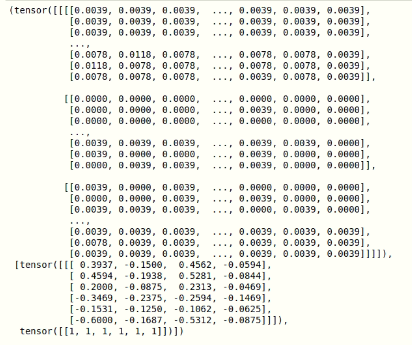# What happens when creating DataBunch from ObjectItemList

``````def get_y_func(o):
print(o.name.replace('.png', '.txt'))
boxes = labels[o.name.replace('.png', '.txt')][:]
boxes = boxes * 640
classes = np.ones((boxes.shape))
print(boxes, classes)
return [boxes, classes]
def get_data():
src = (ObjectItemList.from_folder('/data/aug/images')
.split_by_rand_pct(valid_pct=0.1, seed=233)
.label_from_func(get_y_func))
return src
``````

For example, boxes is now like `[200, 250, 220, 270]`

when I use

``````ww = data.databunch(path='.', bs=1, num_workers=8, collate_fn=bb_pad_collate)
ww.one_batch()
``````I found that the boxes coordinates have become negative.
I wonder if there’s a way to make bounding boxes like `[200, 250, 220, 270] / 640.0` (positive, and relative coordinates)

The box coordinates have been normalized from -1 to 1 because that’s how PyTorch represents points internally (for our use of data augmentation).
So -1,-1 is the top left corner and 1,1 is the bottom right corner.

I understand this, but is there a way to make it normalize to 0 to 1 ?

You have to post-process it then.

1 Like

Yeah… I mean under such structure, how do I post-process the databunch?

``````Data = (ObjectItemList.from_folder('/data/aug/images')
.split_by_rand_pct(valid_pct=0.1, seed=233)
.label_from_func(get_y_func)
learn1 = Learner(Data, model, opt_func=optim.SGD, loss_func=Loss)
``````

Sorry if it’s a stupid question, but I’ve spent hours digging in the docs, plz help…
How do I operate on a DataBunch?

1 Like

You can either add a dataloader transform that can do this operation or do it in your loss function.

1 Like

Thx sgugger! I’m trying with https://docs.fast.ai/basic_data.html#DataBunch.add_tfm and it seems promising!

for future reference, the tfms can be something like:

``````def tfmm(batch):
batch = [(x + 1) * 0.5 for x in batch]
return batch